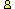CTK Exchange CTK Wiki Math Front Page Movie shortcuts Personal info Awards Terms of use Privacy Policy Cut The Knot! MSET99 Talk Games & Puzzles Arithmetic/Algebra Geometry Probability Eye Opener Analog Gadgets Inventor's Paradox Did you know?... Proofs Math as Language Things Impossible My Logo Math Poll Other Math sit's Guest book News sit's Recommend this siteCTK Exchange

 Subject: "divisibility" Previous Topic | Next Topic
 ConferencesThe CTK ExchangeThis and thatTopic #992Printer-friendly copyEmail this topic to a friend Reading Topic #992
ranjitr303
Member since Nov-23-10
May-11-11, 01:36 PM (EST)"divisibility"

 if (A + mX)/(5 or 10)is divisible by m, then A is divisible by m. also if (A - mX)/(5 or 10)is divisible by m, then A is divisible by m.eg. is 273 divisible by 7?ans; to obtain a number close to 273 that is divisible either by 5 or 10 , we can take X= 1 as m=7. then A+mX = 273+7*1=280 WHICH IS DIVISIBLE BY 10. THEREFORE 273 is divbisible by 7.ranjitr

alexbCharter Member
2806 posts
May-11-11, 01:38 PM (EST)1. "RE: divisibility"
In response to message #0

 >if (A + mX)/(5 or 10)is divisible by m, then A is divisible >by m. >also if (A - mX)/(5 or 10)is divisible by m, then A is >divisible by m. >>eg. is 273 divisible by 7? >ans; to obtain a number close to 273 that is divisible >either by 5 or 10 , we can take X= 1 as m=7. then A+mX = >273+7*1=280 WHICH IS DIVISIBLE BY 10. You probably meant to say that 280 is divisible by 7, right?THEREFORE 273 is >divbisible by 7.

 Conferences | Forums | Topics | Previous Topic | Next Topic
 Select another forum or conference Lobby The CTK Exchange (Conference)   |--Early math (Public)   |--Middle school (Public)   |--High school (Public)   |--College math (Public)   |--This and that (Protected)   |--Guest book (Protected)   |--Thoughts and Suggestions (Public) Educational Press (Conference)   |--No Child Left Behind (Public)   |--Math Wars (Public)   |--Mathematics and general education (Public)You may be curious to have a look at the old CTK Exchange archive.